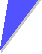# Exercise 2.1-1

## Quick Questions to:

### 2.1 Basic Band TheoryHere are a few quick questions to 2.1.1: Essentials of the Free Electron GasWhat happens, if you do not choose U = U0 = 0 but U = U1 ?What does the sentence "...a plane wave with amplitude (1/L)3/2 moving in the direction of the wave vector k" mean"? Wave vectors, after all, are defined in reciprocal space with a dimension 1/cm. What, exactly, is their direction in real space?Recount what you know bout the spin of an electron.Where does the (1/L)3/2 in the solution of the Schrödinger equation come from? What would one expect for a crystal with the dimension Lx, Ly, Lz?What kind of information is contained in the wave vector k?Consider a system with some given energy levels (including possibly energy continua). Distribute a number N of classical particles, of Fermions and of Bosons, respectively, on these levels. Describe the basic principles employed..How does one always derive the density of states D(E)?Here are a few quick questions to 2.1.2: Diffraction of Electron WavesConsider a fcc and bcc lattice with lattice constant a = 0.3 nm. Give the distance between {100} planes and the distance between the corresponding atomic planes. Do the same thing for the {111} plane of a fcc lattice with just one atom in the base, and for a diamond structure.Remember the Ewald construction? Describe and explain for what kind of situations it is particularly useful.Compare the free electron gas model with and without diffraction.Here are a few quick questions to 2.1.3: Energy Gaps and General Band StructureDraw a one-dimensional realistic periodic potential Now draw in the first Fourier component. Add the probability densities for finding electrons with k = kBZ. Explain the energy splitting and why DE is approximately given by the first Fourier component of the potential.Solution2.1.1 Essentials of the Free Electron Gas2.1.2 Diffraction of Electron WavesSolution to Quick Questions to: 2.1 Basic Band Theory

© H. Föll (Semiconductors - Script)